梯度图像处理_梯度数字图像处理 - CSDN

• 图像处理之图像梯度效果 基本思想： 利用X方向与Y方向分别实现一阶微分，求取振幅，实现图像梯度效果。关于如何计算图像 一阶微分参见这里：http://blog.csdn.net/jia20003/article/details/7562092 使用的两...
图像处理之图像梯度效果

基本思想：
利用X方向与Y方向分别实现一阶微分，求取振幅，实现图像梯度效果。关于如何计算图像
一阶微分参见这里：http://blog.csdn.net/jia20003/article/details/7562092
使用的两种微分算子分别为Prewitt与Sobel，其中Soble在X, Y两个方向算子分别为：Prewitt在X, Y方向上梯度算子分别为：二：程序思路及实现
梯度滤镜提供了两个参数：
– 方向，用来要决定图像完成X方向梯度计算， Y方向梯度计算，或者是振幅计算
– 算子类型，用来决定是使用sobel算子或者是prewitt算子。
计算振幅的公式可以参见以前《图像处理之一阶微分应用》的文章

三：运行效果
原图像如下：基于Prewitt与sobel算子的XY方向振幅效果如下：该滤镜的源代码如下：

package com.process.blur.study;

import java.awt.image.BufferedImage;
/**
*
* @author gloomy-fish
* @date 2012-06-11
*
* prewitt operator
* X-direction
* -1, 0, 1
* -1, 0, 1
* -1, 0, 1
*
* Y-direction
* -1, -1, -1
*  0,  0,  0
*  1,  1,  1
*
* sobel operator
* X-direction
* -1, 0, 1
* -2, 0, 2
* -1, 0, 1
*
* Y-direction
* -1, -2, -1
*  0,  0,  0
*  1,  2,  1
*
*/
public class GradientFilter extends AbstractBufferedImageOp {

// prewitt operator
public final static int[][] PREWITT_X = new int[][]{{-1, 0, 1}, {-1, 0, 1}, {-1, 0, 1}};
public final static int[][] PREWITT_Y = new int[][]{{-1, -1, -1}, {0,  0,  0}, {1,  1,  1}};

// sobel operator
public final static int[][] SOBEL_X = new int[][]{{-1, 0, 1}, {-2, 0, 2}, {-1, 0, 1}};
public final static int[][] SOBEL_Y = new int[][]{{-1, -2, -1}, {0,  0,  0}, {1,  2,  1}};

// direction parameter
public final static int X_DIRECTION = 0;
public final static int Y_DIRECTION = 2;
public final static int XY_DIRECTION = 4;
private int direction;
private boolean isSobel;

direction = XY_DIRECTION;
isSobel = true;
}

public void setSoble(boolean sobel) {
this.isSobel = sobel;
}

public int getDirection() {
return direction;
}

public void setDirection(int direction) {
this.direction = direction;
}

@Override
public BufferedImage filter(BufferedImage src, BufferedImage dest) {
int width = src.getWidth();
int height = src.getHeight();

if (dest == null )
dest = createCompatibleDestImage( src, null );

int[] inPixels = new int[width*height];
int[] outPixels = new int[width*height];
getRGB( src, 0, 0, width, height, inPixels );
int index = 0, index2 = 0;
double xred = 0, xgreen = 0, xblue = 0;
double yred = 0, ygreen = 0, yblue = 0;
int newRow, newCol;
for(int row=0; row<height; row++) {
int ta = 255, tr = 0, tg = 0, tb = 0;
for(int col=0; col<width; col++) {
index = row * width + col;
for(int subrow = -1; subrow <= 1; subrow++) {
for(int subcol = -1; subcol <= 1; subcol++) {
newRow = row + subrow;
newCol = col + subcol;
if(newRow < 0 || newRow >= height) {
newRow = row;
}
if(newCol < 0 || newCol >= width) {
newCol = col;
}
index2 = newRow * width + newCol;
tr = (inPixels[index2] >> 16) & 0xff;
tg = (inPixels[index2] >> 8) & 0xff;
tb = inPixels[index2] & 0xff;

if(isSobel) {
xred += (SOBEL_X[subrow + 1][subcol + 1] * tr);
xgreen +=(SOBEL_X[subrow + 1][subcol + 1] * tg);
xblue +=(SOBEL_X[subrow + 1][subcol + 1] * tb);

yred += (SOBEL_Y[subrow + 1][subcol + 1] * tr);
ygreen +=(SOBEL_Y[subrow + 1][subcol + 1] * tg);
yblue +=(SOBEL_Y[subrow + 1][subcol + 1] * tb);
} else {
xred += (PREWITT_X[subrow + 1][subcol + 1] * tr);
xgreen +=(PREWITT_X[subrow + 1][subcol + 1] * tg);
xblue +=(PREWITT_X[subrow + 1][subcol + 1] * tb);

yred += (PREWITT_Y[subrow + 1][subcol + 1] * tr);
ygreen +=(PREWITT_Y[subrow + 1][subcol + 1] * tg);
yblue +=(PREWITT_Y[subrow + 1][subcol + 1] * tb);
}
}
}

double mred = Math.sqrt(xred * xred + yred * yred);
double mgreen = Math.sqrt(xgreen * xgreen + ygreen * ygreen);
double mblue = Math.sqrt(xblue * xblue + yblue * yblue);
if(XY_DIRECTION == direction)
{
outPixels[index] = (ta << 24) | (clamp((int)mred) << 16) | (clamp((int)mgreen) << 8) | clamp((int)mblue);
}
else if(X_DIRECTION == direction)
{
outPixels[index] = (ta << 24) | (clamp((int)yred) << 16) | (clamp((int)ygreen) << 8) | clamp((int)yblue);
}
else if(Y_DIRECTION == direction)
{
outPixels[index] = (ta << 24) | (clamp((int)xred) << 16) | (clamp((int)xgreen) << 8) | clamp((int)xblue);
}
else
{
// as default, always XY gradient
outPixels[index] = (ta << 24) | (clamp((int)mred) << 16) | (clamp((int)mgreen) << 8) | clamp((int)mblue);
}

// cleanup for next loop
newRow = newCol = 0;
xred = xgreen = xblue = 0;
yred = ygreen = yblue = 0;

}
}
setRGB(dest, 0, 0, width, height, outPixels );
return dest;
}

public static int clamp(int value) {
return value < 0 ? 0 : (value > 255 ? 255 : value);
}

}

转载文章请务必注明出自本博客！
展开全文• 一、图像梯度的定义 图像函数f(x,y)在点(x,y)的梯度是一个具有大小和方向的矢量，设为Gx 和 Gy 分别表示x方向和y方向的梯度，这个梯度的矢量可以表示为： 这个矢量的幅度为 方向角为...

一、图像梯度的定义

图像函数f(x,y)在点(x,y)的梯度是一个具有大小和方向的矢量，设为Gx 和 Gy 分别表示x方向和y方向的梯度，这个梯度的矢量可以表示为：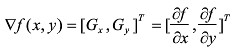这个矢量的幅度为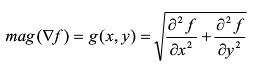方向角为：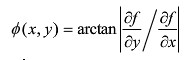对于数字图像而言，相当于对二维离散函数求梯度，如下：

G(x,y) = dx(i,j) + dy(i,j);

dx(i,j)  = I(i+1,j) - I(i,j);

dy(i,j)  = I(i,j+1) - I(i,j);

数字图像中，更多的使用差分来近似导数，最简单的梯度近似表达式如下：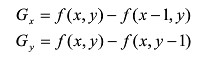梯度的方向是函数f(x,y)变化最快的方向，当图像中存在边缘时，一定有较大的梯度值，相反，当图像中有比较平滑的部分时，灰度值变化较小，则相应的梯度也较小，图像处理中把梯度的模简称为梯度，由图像梯度构成的图像成为梯度图像(we can get the gradient image through computing the gradient of each pixel in the image)

经典的图像梯度算法是考虑图像的每个像素的某个邻域内的灰度变化，利用边缘临近的一阶或二阶导数变化规律，对原始图像中像素某个邻域设置梯度算子，通常我们用小区域模板进行卷积来计算，有Sobel算子、Robinson算子、Laplace算子等。

二、图像一阶微分应用

首先看一下数学中一维的微分公式Δf = f(x+1) – f(x), 对于一幅二维的数字图像f(x,y)而言，需要完

成XY两个方向上的微分，所以有如下的公式：分别对X,Y两个方向上求出它们的偏微分，最终得到梯度Delta F.

对于离散的图像来说,一阶微分的数学表达相当于两个相邻像素的差值，根据选择的梯度算

子不同，效果可能有所不同，但是基本原理不会变化。最常见的算子为Roberts算子，其它

常见还有Sobel，Prewitt等算子。以Roberts算子为例的X,Y的梯度计算演示如下图：图像微分(梯度计算)是图像边缘提取的重要的中间步骤，根据X,Y方向的梯度向量值，可以

得到如下两个重要参数振幅magnitude, 角度theta，计算公式如下：magnitude表示边缘强度信息

theta预言边缘的方向走势。

假如对一幅数字图像，求出magnitude之后与原来每个像素点对应值相加，则图像边缘将被

大大加强，轮廓更加明显，是一个很典型的sharp filter的效果。


展开全文• 前面我们介绍过图像梯度，其定义是根据微积分的定义在二维离散函数中推导出来的。但是，我们需要理解，梯度只是一个工具，方法，核心目的是得到像素点与其相邻像素的灰度值变化情况，并通过这种变化来增强图像。...
前面我们介绍过图像的梯度，其定义是根据微积分的定义在二维离散函数中推导出来的。但是，我们需要理解，梯度只是一个工具，方法，核心目的是得到像素点与其相邻像素的灰度值变化情况，并通过这种变化来增强图像。这种原始定义的梯度只是这种灰度值变化情况的度量工具。
我们再回顾一下，假设某像素与其8领域用如下矩阵表示：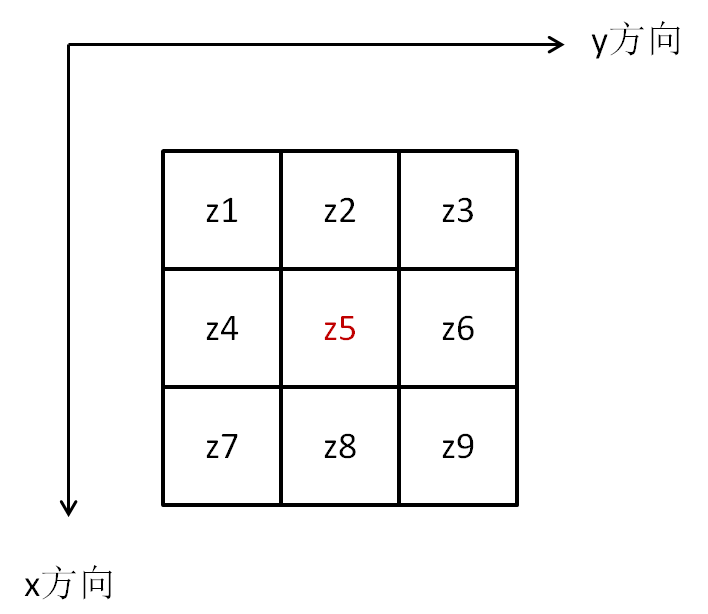那么，根据图像梯度的定义：
gx  =  z8 - z5
gy  =  z6 - z5
上面提到，这种原始定义的梯度只是这种灰度值变化情况的度量工具，这种度量工具只有这一种吗？显然不是的！
z9-z5算不算灰度值的变化？z1-z5呢？z7-z5呢？z4-z5呢？z3-z5呢？
我们利用梯度的本质目的，是要找到某像素与其相邻像素的灰度差值，并放大这种差值，从而用于图像增强。而原始定义的梯度计算方法只是灰度差值计算方法中的一种，还可以有其它计算方法来度量这种变化。
为简化起见，我们把这些计算方法统称为梯度算子。根据不同的相邻像素差值计算得到的效果可能有所不同，但基本原理是一致的。
例如罗伯特（Roberts）交叉梯度算子，定义的是：
gx = z9-z5
gy = z8-z6
为什么名字中有“交叉”，看看下图就知道了。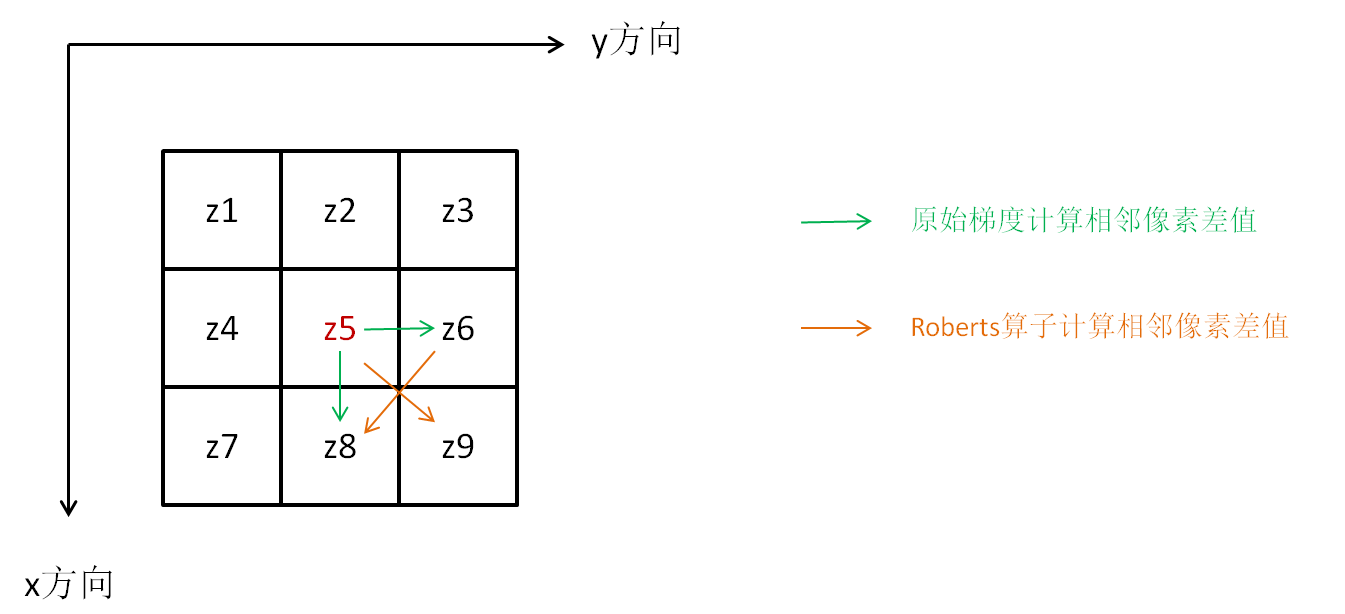我们可以看到，不管是原始梯度也好，Roberts算子也好，都只是利用了z5,z6,z8,z9的像素值，那么可不可以扩展到8领域呢，当然是可以的。
例如，你可以自己定义一个：
gx = (z7+z8+z9)-(z1+z2+z3)
gy = (z3+z6+z9)-(z1+z4+z7)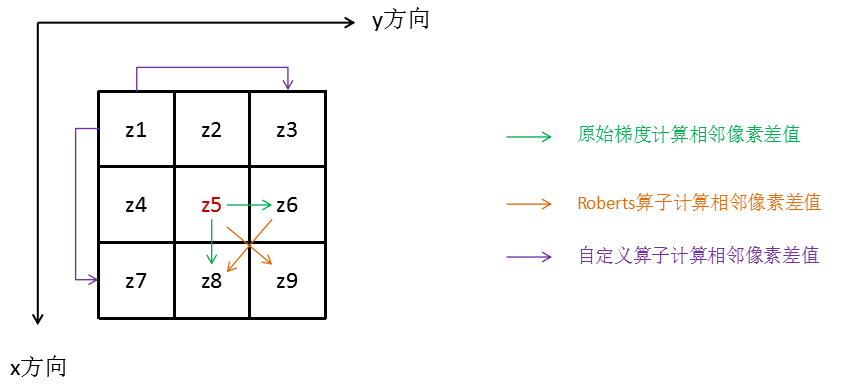事实上，这个你自定义的算子和著名的Sobel算子非常接近了，只是Sobel算子增加了距离权重而已。Sobel算子的定义如下（与中心点Z5更近的点Z3，Z4，Z6，Z8的权重为2，其它对角线上的权重为1）：
gx = (z7+2*z8+z9)-(z1+2*z2+z3)
gy = (z3+2*z6+z9)-(z1+2*z4+z7)
关于Sobel算子后面还会再重点介绍，下面用Roberts交叉算子来看看图像增强的效果。

import cv2
import numpy as np

row, column = moon.shape
moon_f = np.copy(moon)
moon_f = moon_f.astype("float")

Roberts = np.zeros((row, column))

for x in range(row - 1):
for y in range(column - 1):
gx = abs(moon_f[x + 1, y + 1] - moon_f[x, y])
gy = abs(moon_f[x + 1, y] - moon_f[x, y + 1])
Roberts[x, y] = gx + gy

sharp = moon_f + Roberts
sharp = np.where(sharp < 0, 0, np.where(sharp > 255, 255, sharp))
sharp = sharp.astype("uint8")

cv2.imshow("moon", moon)
cv2.imshow("Roberts_sharp", sharp)
cv2.waitKey()123456789101112131415161718192021222324
输出结果：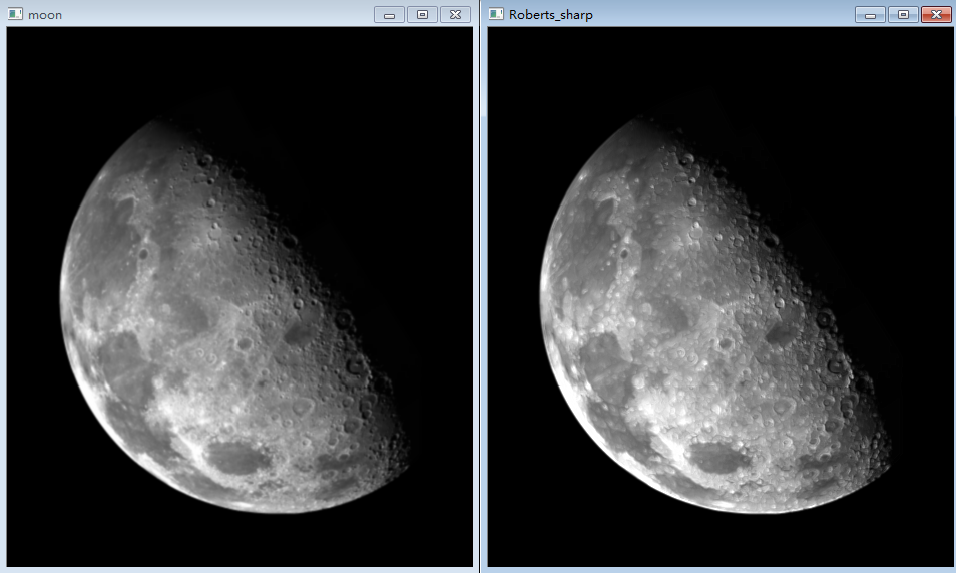再看看用上面自定义算子的情况：
user_defined = np.zeros((row, column))

for x in range(1, row - 1):
for y in range(1, column - 1):
gx = abs((moon_f[x + 1, y - 1] + moon_f[x + 1, y] + moon_f[x + 1, y + 1]) - (
moon_f[x - 1, y - 1] + moon_f[x - 1, y] + moon_f[x - 1, y + 1]))
gy = abs((moon_f[x - 1, y + 1] + moon_f[x, y + 1] + moon_f[x + 1, y + 1]) - (
moon_f[x - 1, y - 1] + moon_f[x, y - 1] + moon_f[x + 1, y - 1]))

user_defined[x, y] = gx + gy

sharp = moon_f + user_defined
sharp = np.where(sharp < 0, 0, np.where(sharp > 255, 255, sharp))
sharp = sharp.astype("uint8")

cv2.imshow("moon", moon)
cv2.imshow("defined_sharp", sharp)
cv2.waitKey()
1234567891011121314151617181920
输出结果：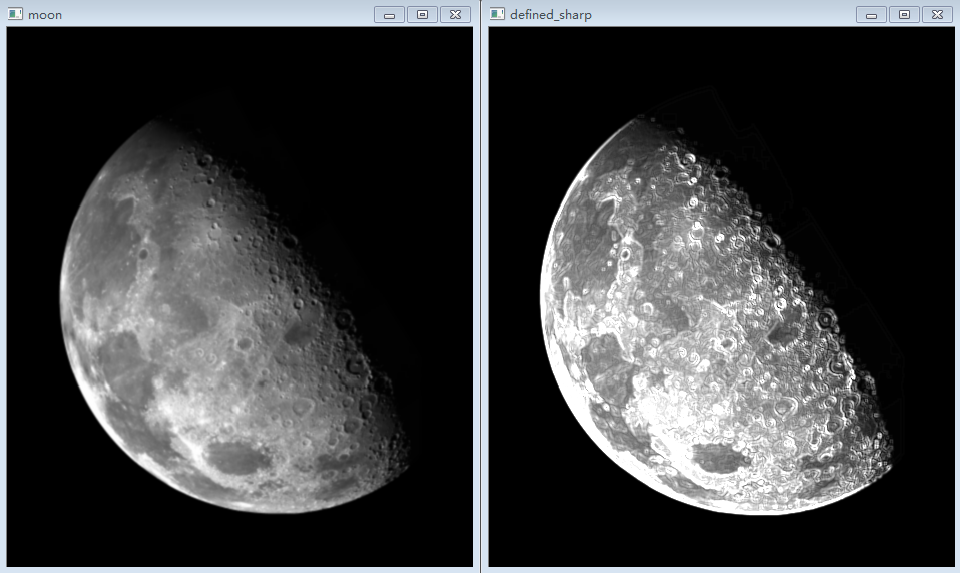转载自：https://blog.csdn.net/saltriver/article/details/78987170


展开全文• 图像在计算机中以数字图像的形式存储，即以数值矩阵的形式存在，形成了离散的数值信号，在此基础上，对于图像处理中的数值的多样性计算分析也影响着初步图像分析。1.图像梯度的定义图像函数f(x,y)在点(x,y)的梯度是...
图像在计算机中以数字图像的形式存储，即以数值矩阵的形式存在，形成了离散的数值信号，在此基础上，对于图像处理中的数值的多样性计算分析也影响着初步图像分析。图像梯度的定义：图像函数f(x,y)在点(x,y)的梯度是一个具有大小和方向的矢量，设为Gx 和 Gy 分别表示x方向和y方向的梯度，这个梯度的矢量可以表示为：图像梯度：G(x,y)=dx i +dy j;dx(i,j)=Img(i+1,j)-Img(i,j);dy(i,j)=Img(i,j+1)-I(i,j);Img(i,j)是图像数值矩阵的像素值，（i，j）为像素相应坐标。Gradient(Img)函数求的是数值上的梯度，假设Img为图片数值矩阵.Matlab中计算方法: [Rx,Ry]=gradient(Img)；(1)水平方向梯度Rx，Rx的第一列元素为原矩阵第二列与第一列元素之差，Rx的第二列元素为原矩阵第三列与第一列元素之差除以2，以此类推：Fx(i,j)=(F(i,j+1)-F(i,j-1))/2。最后一列则为最后两列之差。(2)垂直方向梯度Ry，同理，可以得到Fy。
展开全文• ## 图像梯度的基本原理

万次阅读 多人点赞 2018-01-06 10:37:22
前面我们提到，当用均值滤波器降低图像噪声的时候，会带来图像模糊的副作用。我们当然希望看到的是清晰图像。那么，清晰图像和模糊图像之间的差别在哪里呢？从逻辑上考虑，图像模糊是因为图像中物体的轮廓不明显，...图像处理 opencv 图像梯度
• 前面我们提到，当用均值滤波器降低图像噪声的时候，会带来图像模糊的副作用。我们当然希望看到的是清晰图像。那么，清晰图像和模糊图像之间的差别在哪里呢？从逻辑上考虑，图像模糊是因为图像中物体的轮廓不明显，...
• ## 图像处理中的梯度

万次阅读 2017-08-19 14:19:50
由于图像在计算机中以数字图像的形式进行 存储， 即图像是离散的数字信号， 对数字图像梯度使用差分来代替连续信号中 的微分。 由于图像在计算机中以数字图像的形式进行 存储， 即图像是离散的数字信号， ...
• 学过图像处理的人，对图像梯度的概念应该都不陌生，图像梯度，可以将一个图像值变成一个向量，如下图所示： 简单来说，就是对像素值，在 x, y 方向进行求导，从而可以得到图像在 x, y 方向的梯度梯度域的图像处理...
• 介绍图像处理形态学基本操作腐蚀与膨胀组合实现图像基本梯度计算、内部梯度、外部梯度与方向梯度等扩展知识点。OpenCV 图像梯度 膨胀 图像处理
• 下面简要介绍一下不同的梯度算子对于图像处理的区别： 其中Roberts算子，又称罗伯茨算子，是一种最简单的算子，是一种利用局部差分算子寻找边缘的算子。他采用对角线方向相邻两象素之差近似梯度幅值检测边缘。检测...MATLAB
• 基本思想：利用X方向与Y方向分别实现一阶微分，求取振幅，实现图像梯度效果。使用的两种微分算子分别为Prewitt与Sobel，其中Soble在X, Y两个方向算子分别为：Prewitt在X, Y方向上梯度算子分别为：二：程序思路及实现...
• 人眼区域分割（2） 在人眼区域分割（1）中,对人脸图片求水平投影曲线（也叫IPF）,其反映的是每行像素和的变化曲线，即反应了整体的灰度变化，并没有反映眼睛...梯度积分投影 分析投影曲线 人眼区域分割 总结 ...
• 其实，梯度下降算法数学本质上是一个求解最优解的一个方法，但应用到数学图像处理中的话就有了他的物理意义，即从起始点到目的点的迭代次数最少的算法。 从起始点，以步长为半径，对损失函数求一阶导数（梯度）找到...图像处理
• ## 图像梯度域融合

千次阅读 2015-11-10 17:44:48
图像梯度域融合 图像梯度域重建 图像泊松编辑 混合梯度融合 彩色图像灰度化的问题 图像梯度域重建-原理 该问题中心思想是利用梯度实现灰度图像的重建。为后面的问题作铺垫。 该问题用到了梯度与最小二乘的思想。 ...
• clear all clc I=imread('kids.tif') ...//梯度处理水平方向特征明显 B2=[-1 0 1;-1 0 -1;-1 0 -1];//梯度处理竖直方向特征明显 I1=I; I2=I; temp=zeros(3,3);//采用3x3的模板进行处理 for i=2:M-1 for j=...
• 首先要知道梯度是个什么概念，在数学中我们知道梯度是一个向量，函数在一点的梯度在数学上它是这么定义的：它的方向是函数在这点的方向导数取得最大值的方向，它的模就等于方向导数的最大值。  梯度经常用...图像梯度
• 结合对图像处理的学习和实践，大致总结了一部分图像处理研究中所需的数学原理基础。这些内容主要涉及微积分、向量分析、场论、泛函分析、偏微分方程、复变函数、变分法等。总结、归纳、提取了上面这些数学课程中，在...图像处理 信号处理 图像
• 在数字图像处理过程中，经常会遇到求梯度后，重新构建图像的问题。一般情况下，都是通过解泊松方程(还有其他方式重构图像，具体算法如下图所示,)，利用拉普拉斯算子求解；但有一点请注意泊松方程求出的只是近似值，...图像处理 梯度 图像重建
• ## 从梯度场重建图像

千次阅读 2015-06-25 14:32:19
很多图像处理的算法是在图像的梯度域完成的。比如图像增强、图像的融合、图像的边缘检测和分割等。在这些算法中或者一部分或者全部在梯度域中完成。  图像梯度的概念很简单。图像梯度可以把图像看成二维离散函数，...
...